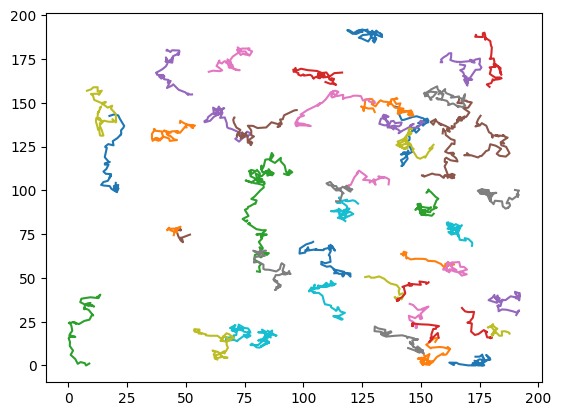This tutorial demonstrates how to generate fluorescence microscopy videos of the AnDi trajectories using the function `transform_to_video`.

## 1. Setup

Importing the dependencies needed to run this tutorial.

``````import numpy as np
import random
import imageio
import matplotlib.pyplot as plt
import deeptrack as dt
from andi_datasets.models_phenom import models_phenom``````

## 2. Defining example diffusion model

As an example, We generate the trajectories of dimerization model from `models_phenom`.

### 2.1. Dimerization

Defining simulation parameters.

``````T = 50 # number of time steps (frames)
N = 50 # number of particles (trajectories)
L = 1.5 * 128 # length of the box (pixels) -> extending fov by 1.5 times
D = 0.1 # diffusion coefficient (pixels^2/frame)``````
``````trajs, labels = models_phenom().dimerization(
N=N,
L=L,
T=T,
alphas=[1.2, 0.7],
Ds=[10 * D, 0.1 * D],
r=1,  # radius of the particles
Pb=1,  # binding probability
Pu=0,  # unbinding probability
)``````

Plotting trajectories.

``````for traj in np.moveaxis(trajs, 0, 1):
plt.plot(traj[:,0], traj[:,1])
plt.show()``````## 3. Generating videos

### 3.1. Import functions

For generating videos we import `transform_to_video` function from `andi_datasets` package. Additionally we import `play_video` function to display the videos within the jupyter notebook.

``from andi_datasets.utils_videos import transform_to_video, play_video``

### 3.2. Usage

The trajectory data generated can be directly passed through `transform_to_video` to generate fluorescence videos of the particles.

#### 3.2.1. Generating a sample video

``````video = transform_to_video(
trajs,
)``````
``play_video(video)``